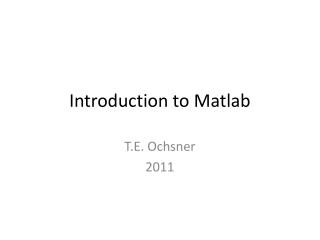Download PresentationIntroduction to Matlab

# Introduction to Matlab - PowerPoint PPT PresentationDownload Presentation## Introduction to Matlab

- - - - - - - - - - - - - - - - - - - - - - - - - - - E N D - - - - - - - - - - - - - - - - - - - - - - - - - - -
##### Presentation Transcript

1. Introduction to Matlab T.E. Ochsner 2011

2. Getting Started with Matlab • http://www.mathworks.com/videos/matlab/getting-started-with-matlab.html

3. OSU Matlab Site License • E-mail Gary Hoefar in the IT Dep. gary.hoefar@okstate.edu • Request access to download and activate Matlab. • After you get Gary’s response, go to http://www.mathworks.com/ • Create an account using the same name and e-mail you gave Gary. • Download, install, and activate the appropriate version for your operating system • Current release is R2011a

4. Weak traceability Record data in field or lab book Import the transformed data into SigmaPlot Enter data in Excel Enter some parameters in Excel Create some figures in SigmaPlot Perform some calculations Copy and paste the SigmaPlot figures in your thesis Enter the SAS output into a table in your thesis Copy and paste the tranformed data into a convenient format for SAS Import the transformed data to SAS and do some more calculations

5. Improved traceability Record data in field or lab book Create raw data file in .csv, .xlsx, or other format Create Matlab script to process the data, generate figs, and run stats. Copy and paste the Matlab figures and stats into your thesis.

6. Weak graphics

7. Improved graphics

8. Customization function theta_vg = vangenuchten(vgparams, matric) %VANGENUCHTEN returns the estimated water content vector corresponding to %the input vectors containing the matric potential and the function %parameters. The units of the matric potential and the units of the %parameter "alpha" must cancel. theta_s = vgparams(1); %saturated water content theta_r = vgparams(2); %residual water content n = vgparams(3); %shape factor alpha = vgparams(4); % inverse of air entry potential %m = 1 - 1/n; m = vgparams(5); theta_vg = ((1+(-alpha*matric).^n).^(-m))*(theta_s - theta_r) + theta_r;

9. Efficiency and Speed • Excel spreadsheet with 106 data points = 8.8 Mb • Matlab data file with 106 data points = 0.4 Mb • “Consider the problem of inverting the covariance matrix of a first-order autoregressive process with dimension n=1000 and correlation parameter 0.5 and unit innovation variance…. PC with a 400  MHz Pentium II  processor running WinNT with 256 MB RAM.” http://www.stats.uwo.ca/faculty/aim/epubs/MatrixInverseTiming/default.htm

10. Disadvantages of Matlab • Harder to learn than Excel • Weaker on statistics than SAS • For example: no built-in “repeated measures” procedure